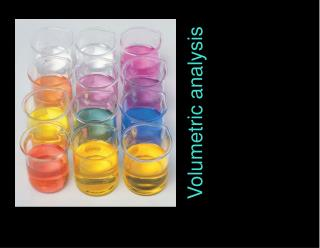DownloadDownload PresentationVolumetric analysis

Volumetric analysis

Télécharger la présentationVolumetric analysis

- - - - - - - - - - - - - - - - - - - - - - - - - - - E N D - - - - - - - - - - - - - - - - - - - - - - - - - - -
Presentation Transcript

1. Volumetric analysis

2. A very useful method of chemical analysis is based upon determining the volume of a solution of known concentration that is needed to react exactly with the substance being analysed. This is called volumetric analysis. It uses molarity and calculations based upon that concept to work out answers (as was discussed on pp. 259–63 of Chemistry in Use Book 1). The process of determining the volume of one reactant solution which exactly reacts with a given amount of another reactant is called titration. Before discussing volumetric analysis, we need first to consider some preliminary matters, such as equivalence point and indicators.

3. The commonest indicators are acid–base indicators. They change colour as pH changes. The fi rst indicator was litmus, a dye that was extracted from lichens which are mixed algae–fungi organisms that grow on rocks and trees. Litmus is red in acid solution (pH < 6) and blue in alkaline solution (pH > 8). Between pH 6 and 8 it gradually changes colour from red to blue. We say that litmus has a pH range of 6 to 8, meaning that this is the range of pH over which it changes colour. Many other acid–base indicators have been isolated or synthesised. They are actually weak acids with the conjugate base having a different colour from that of the acid itself. Different indicators have different acid strengths (Sections 1.16 (pp. 224–5) and 2.9 (pp. 254–6)); and so they change colours over different pH ranges. Figure 3.1 shows the colours of four indicators in acidic, neutral and alkaline solutions. Common indicators are shown in Table 3.1 along with their colour changes and their pH ranges. Figure 3.2 shows t

4. 3.3 pH METERS pH meters are instruments that measure pH directly. The sensing device, shown in Figure 3.3, consists of a pair of electrodes (often mounted concentrically in the one unit). The measuring electrode, called a glass electrode, consists of a very thin and fragile membrane made out of a special kind of glass. This develops an electrical potential which depends upon pH. The other electrode is a reference electrode. When this sensing unit is immersed in the solution to be tested, it forms a galvanic cell; the accompanying electronics unit simply measures the voltage (EMF) of this cell and converts it into a pH reading. A big advantage of a pH meter is that in measuring the pH of a solution, it does not alter the pH of the solution in any way; it does not disturb any chemical equilibria involved.